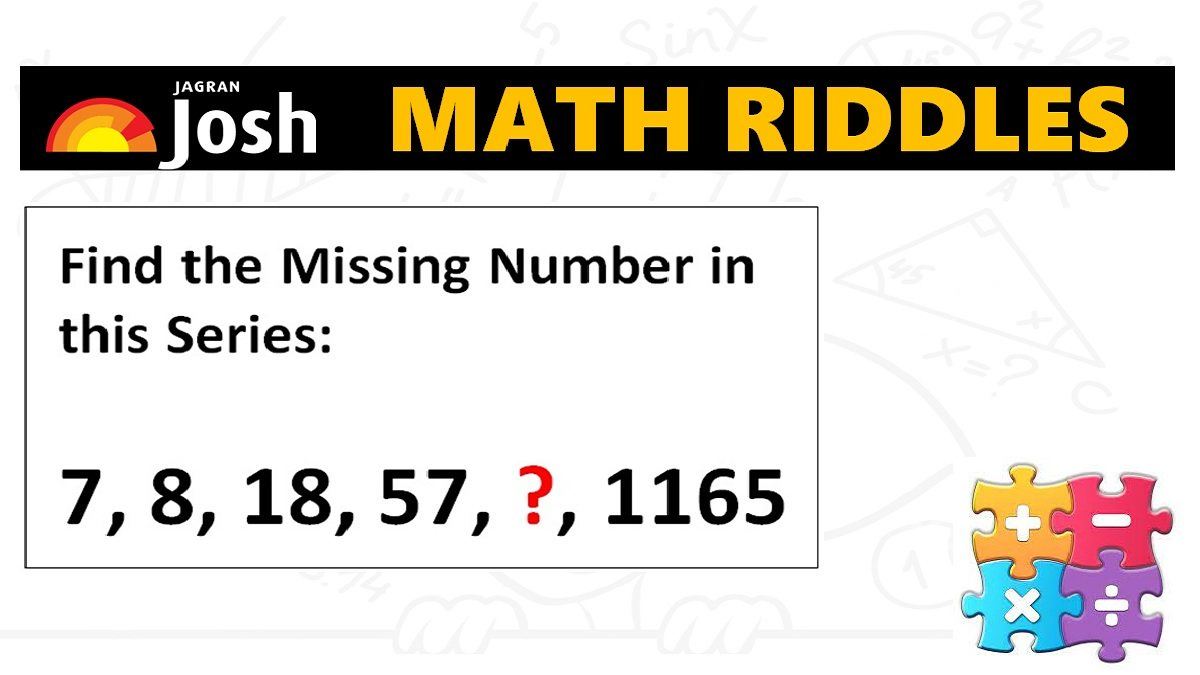# Math Riddles: Can You Find The Next Number In These Math Puzzle Series?

Math Riddles Challenge: You are 100% genius if you can find the missing numbers in these mathematics puzzle in less than 1 minute each?Math Riddles: Can You Find The Next Number In These Math Puzzle Series?

Math Riddles: Check out some brain power mathematics puzzles that 99 percent of people could not solve. You have to find the missing numbers in these math number series. These are some tricky logical math puzzles, so you have to unlock the logic behind them to find the correct answer. You have exactly 1 minute to solve each puzzle. These math problems are a booster in the preparation for government exams, banking, civil services aptitude and logical reasoning test. Let us begin some brain exercises.

## Solution

### Maths Logic Puzzle #1

Explanation:

7, 8, 18, 57, ?, 1165

=> 7 x 1 + 1 = 7 + 1 = 8

=> 8 x 2 + 2 = 16 + 2 = 18

=> 18 x 3 + 3 = 54 + 3 = 57

=> 57 x 4 + 4 = 228 + 4 = 232

We can also cross-check by applying the same logic and see if we are getting 1165 as in the problem.

=> 232 x 5 + 5 = 1160 + 5 = 1165

Explanation:

## Tell us in comments: Did you solve these mathematics puzzles in less than 1 minute each?

Check out more math puzzles!

Also Read: Math Riddles: Only 1 out 5 People Can Solve These 5-Minute Maths Puzzles

Math Riddles: 5-Minute Maths Puzzles, Find the Answers in Less than 1 Minute

Also Read: Math Riddles: 5-Minute Mathematics Puzzles, 99% Failed! Can You Solve Them?

Also Read: Math Riddles: Only 1% Genius Can Solve These Tricky Mathematics Puzzles in 3 Minutes

Get the latest General Knowledge and Current Affairs from all over India and world for all competitive exams.
खेलें हर किस्म के रोमांच से भरपूर गेम्स सिर्फ़ जागरण प्ले पर